## Disk Covering Problem

N.B. A detailed on-line essay by S. Finch was the starting point for this entry.

Given a Unit Disk, find the smallest Radiusrequired forequal disks to completely cover the Unit Disk. For a symmetrical arrangement with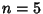(the Five Disks Problem),, whereis the Golden Ratio. However, the radius can be reduced in the general disk covering problem where symmetry is not required. The first few such values are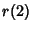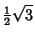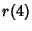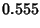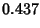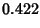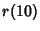Here, values for, 8, 9, 10 were obtained using computer experimentation by Zahn (1962). The valueis equal to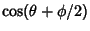, whereandare solutions to(1)(2)(3)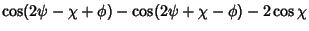(4)
(Neville 1915). It is also given by, whereis the largest real root of(5)

maximized over all, subject to the constraints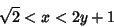(6)(7)

and with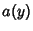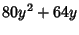(8)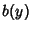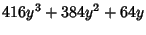(9)(10)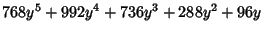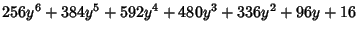(11)(12)(13)

(Bezdek 1983, 1984).

Lettingbe the smallest number of Disks of Radiusneeded to cover a disk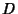, the limit of the ratio of the Area ofto the Area of the disks is given by(14)

(Kershner 1939, Verblunsky 1949).

References

Ball, W. W. R. and Coxeter, H. S. M. The Five-Disc Problem.'' In Mathematical Recreations and Essays, 13th ed. New York: Dover, pp. 97-99, 1987.

Bezdek, K. Über einige Kreisüberdeckungen.'' Beiträge Algebra Geom. 14, 7-13, 1983.

Bezdek, K. Über einige optimale Konfigurationen von Kreisen.'' Ann. Univ. Sci. Budapest Eötvös Sect. Math. 27, 141-151, 1984.

Finch, S. Favorite Mathematical Constants.'' http://www.mathsoft.com/asolve/constant/circle/circle.html

Kershner, R. The Number of Circles Covering a Set.'' Amer. J. Math. 61, 665-671, 1939.

Neville, E. H. On the Solution of Numerical Functional Equations, Illustrated by an Account of a Popular Puzzle and of its Solution.'' Proc. London Math. Soc. 14, 308-326, 1915.

Verblunsky, S. On the Least Number of Unit Circles which Can Cover a Square.'' J. London Math. Soc. 24, 164-170, 1949.

Zahn, C. T. Black Box Maximization of Circular Coverage.'' J. Res. Nat. Bur. Stand. B 66, 181-216, 1962.